Hans Walser, [20090726a]

Regular K-gon, Rectangles, and Trapezoids

We start with a regular K-gon and add similar rectangles on every side. The shape of the rectangles depends on K: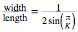Shape of the rectangles

Then we proceed by adding rectangles, as explained in the next example.

The lengths of the different rectangles are in the proportions 1:1:2:3:5:8…, i. e. in the proportions of the Fibonacci-numbers.

2        Example

Starting by a regular yellow pentagon () in the unit circle we add similar rectangles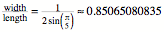on every side. Then we proceed as indicated in the following figure.Pentagon and rectangles

We get red isosceles trapezoids between the white rectangles. In the first ring we see red triangles, but we count them as special red trapezoids with upper side zero.

Remark: There is (of course) a link to the golden section, since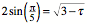.

Dissecting the rectangles into congruent rhombuses, we see that the lengths of the rectangles are 1, 1, 2, 3,  … . These are the Fibonacci Numbers.Congruent rhombuses

We can dissect the triangles and trapezoids into congruent triangles and hexagons (both not regular).Nice figure

3        Modification

We can modify the figure by transforming the white rectangles into parallelograms. This does not change shape or size of the red trapezoids.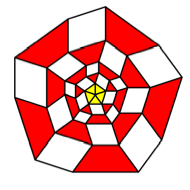Collapsing the rectangles

Most useful is the third of these figures with five sectors.

All these figures can be dissected into parallelograms (instead of rhombuses) and triangles and hexagons. The triangles and hexagons don’t change their shape or size.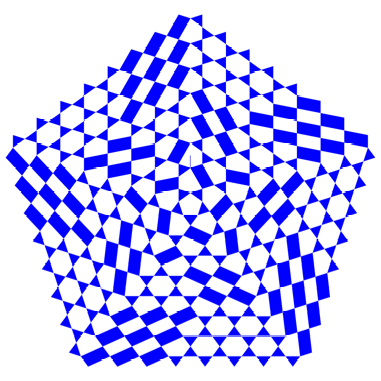Modification

4        Some calculations

We use the notations of the following figure.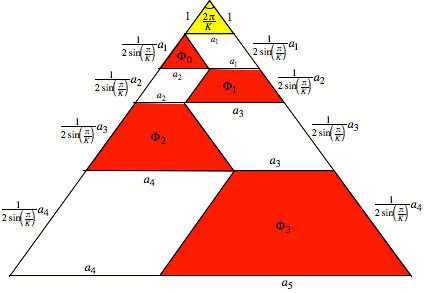Notations

First we get:Studying a red trapezoid, we see:This is the usual Fibonacci recursion. Therefore we have: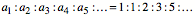For the areasof the red trapezoids we get by some calculations: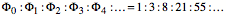These are every second Fibonacci number. The areas of the red trapezoids are in a rational relation.

5        Special cases

5.1      K = 1

Division by zero.

5.2      K = 2

We use rectangles with(half squares).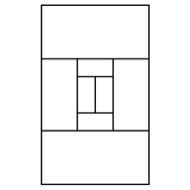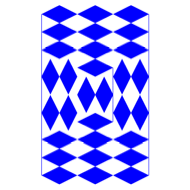K = 2

The entire Figure is a rectangle, which approximates the so called Golden Rectangle with.

5.3      K = 3

We use rectangles with.K = 3

Both figures fit in the same triangular lattice. In this lattice it is easy to check the Fibonacci property.Triangular latticeTriangular latticeDissections

5.4      K = 4

We use rectangles with.  This is the so called DIN format.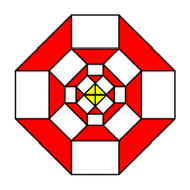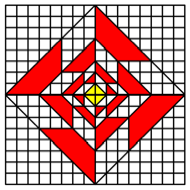K = 4

The figure on the right fits into a square lattice, but not so the figure on the left.Disections

5.5      K = 5

See example in the introduction.

5.6      K = 6

We get “rectangles” with, i. e. squares.K = 6

The figure on the right fits into a regular triangular lattice. The figure on the left not, since rectangles and regular triangles don’t like each other.Triangular lattice

In the dissection of the figure on the left we get squares, equilateral triangles, and regular hexagons. This is the most regular case.Squares, equilateral triangles, and regular hexagons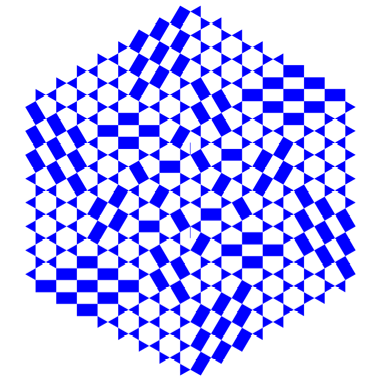Pattern in a hexagon

5.7      K = 7

We use rectangles with. Now the rectangles are staying out like the hairs of Struwwelpeter.K  = 7

5.8      K = 8

Rectangles with.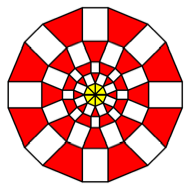K = 8

5.9      K = 10

Rectangles with.  We have golden rectangles.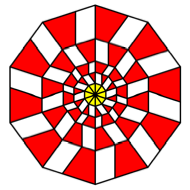K = 10

5.10   K = 30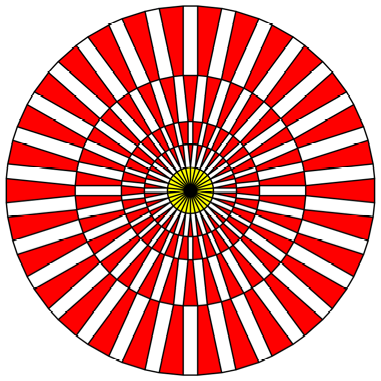K = 30

The figure approximates circles with the radii 1, 2, 3, 5, and 8, i. e. the Fibonacci numbers.Dissection

References

[Deshpande 2009]      Deshpande, M. N. : Proof Without Words: Beyond Extriangles. MATHEMATICS MAGAZINE. Vol. 82, No. 3, June 2009, p. 208.